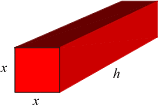index: click on a letter A B C D E F G H I J K L M N O P Q R S T U V W X Y Z A to Z index index: subject areas numbers & symbols sets, logic, proofs geometry algebra trigonometry advanced algebra & pre-calculus calculus advanced topics probability & statistics real world applications multimedia entrieswww.mathwords.com about mathwords website feedback

Right Square Prism

A box with at least one pair of opposite faces that are squares. It can also be described as a right prism with square bases. A right square prism which has square lateral surfaces is a cube.

 Right Square Prism Volume = x2h Lateral Surface Area = 4xh Surface Area = 2x2 + 4xh